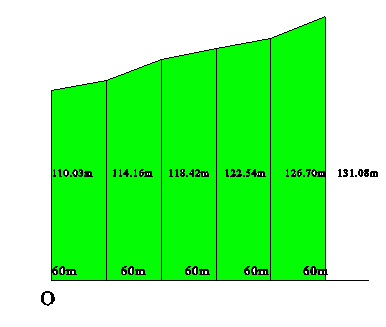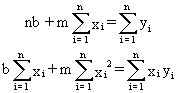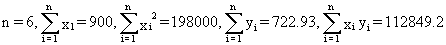# Geomatics: Using Linear Regression to Reposition Survey Pins## Background:

Legal surveying is concerned with the measurement and description of real property. In towns and cities the positions of significant points on property lines are marked with posts and/or pins. It may happen that, through time, the position of the original pins shifts because of ground movement or hedge growth or ......

## Problem:

Over time the posts on the back line of a set of lots have moved and must be re-posted in a straight line. This is accomplished by fitting a straight line through these points using least squares, a statistical technique which minimizes the sum of the squares of the errors between the observed points and the line y = mx + b which is found to best represent the line through these points. In this case all side lines are at right angles to the front line of the lots, all measurements are in metres, and the origin of the system, O, is established at the front, left-hand corner of the first lot.

 x y 0 60 120 180 240 300 110.03 114.16 118.42 122.54 126.7 131.08

All values for x and y are in metres.

To fit a line of the form y = mx + b through these points a least-squares technique is used to find values for m and b. This leads to the following equations in m and b, known as normal equations:where n = the number of points and (xi,yi) are the coordinates of the points.

For these data,The normal equations are:

6b + 900m = 722.93

900b + 198000m = 112849.2

Solving gives b = 109.989, m = 0.07. So the equation of the best-fitting line through these points is y = 0.07x + 109.989.

Using this equation the posts can be repositioned on a straight line as indicated in the table.

 x y 0 60 120 180 240 300 109.99 114.19 118.39 122.59 126.79 130.99Written by Colin Lawrence, June 26, 1998